#### Interference, Physics tutorial

Wave Nature of Light:

It has been found that in reality light is the form of wave traveling in straight lines represented by rays. These waves are different from sound waves as sound waves are longitudinal while light waves vibrate perpendicularly to direction of propagation. Also sound wave is the mechanical wave, while light is the electromagnetic wave. Human eye can see light of wavelength that lies between 450 x 10-9m and 750 x 10-9m. This range of wavelength is called as visible range of electromagnetic spectrum. Few electromagnetic waves have shorter wavelength that visible light, whereas others have longer wavelength then visible light. The invisible electromagnetic waves vary from visible light only in terms of wavelength and in ways by which they are generated.

Coherent Source:

Consider two interfering waves (light waves). If these interfering waves are generated randomly, it signifies that they are out of phase with each other (or there is continual change of phase), then such sources of interfering waves are called as incoherent source.

Coherent sources are sources which should emit light waves of

(i) Same frequency or wavelength and

(ii) The constant phase difference or which are always in phase with each other (and they should be traveling in same or almost same direction).

Interference:

Let two waves originated from two coherent sources of light A and B, of same frequency (or wavelength) travel in the same direction and having the constant phase difference with the passage of time. Resultant intensity of light don't distributed uniformly in space. Thus, non-uniform distribution of the light intensity because of combined effect (superposition) of two waves is known as interference. Generally, it is found that at some points intensity of light is maximum and on other points, intensity of light is minimum.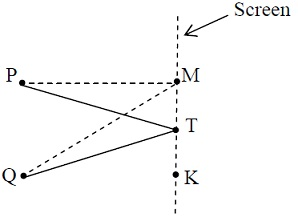There are different kinds of interference patterns. Interference is of two kinds: (i) constructive interference (ii) destructive interference.

(a) Constructive Interference:

Constructive interference happens when effect of two interfering waves is additive at point of interest. At these points, intensity becomes maximum. Resultant vibration at point is attained by adding them (their amplitudes). The bright 'band' of light is attained at these points. That is, they add up to give something bigger than contribution from either source of the two sources. Assume we consider two coherent sources A and B which are exactly in stage, interference effect at the equidistant point T is constructive, and attained by adding displacement of individual wave at the point.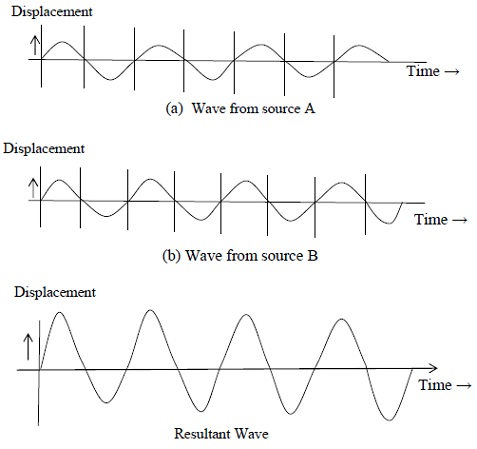In general constructive interference would take place if path difference, d, at any point P is multiples of zero or whole number wavelength that is

d = nλ

Where n = 0, 1, 2 ......... so on. ..........Eq.1

On the other hand destructive interference happens when net effect at the point of interest, is the effect of one source minus the effect of other source.

(b) Destructive Interference:

Destructive interference takes place at some point where intensity is minimum. This is the case in which interference effects is smaller than that generated by individual waves. At these points, dark band of light is obtained. Path difference d for constructive interference can be provided by expression

d = QM - PM = (n + 1/2)λ..........Eq.2

Where n = 0, 1, 2, 3,.........so on

Optical Path:

Let a medium of refractive index m and its thickness is t. The light ray is traveling from the point O (in air) to another point A (in air) through the medium that is introduced in between this ray. So light ray partly travels in air and partially in the medium introduced between. In interference phenomena, optical paths of coherent light rays are found. Product of refractive index m and path length t is known as optical path in medium.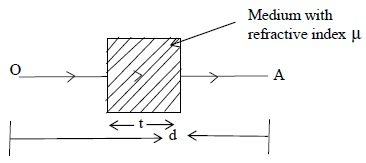Optical path = μt

When the glass slab is introduced between the ray from O to A, then expression for optical path is provided by

Optical path = (d-t) + μt

=d + (μ-1) t ......... (1.3)

This illustrates that effect of introducing the material of thickness t, refractive index m is increased optical path by (μ - 1) t. In other words, air path OA is increased by the amount (μ - 1)t because of introduction of plate of material of refractive index μ and thickness t. Young had first shown the interference of light.

The Young's Double slit experiment:

Two slit A and B are illuminated by the same monochromatic source of light S. This ensures that the wave leaving A and B are coherent.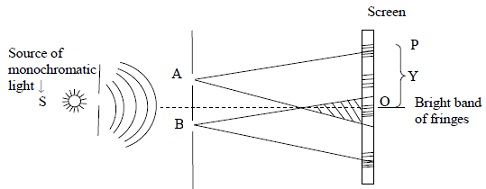It was Thomas Young who first observed that if a transparent screen is placed formed in the region where the beams overlap. At O, along the perpendicular bisector of AB, the waves due to A and B are in phase. Hence, a bright band or fringe is formed. This is because the two light wave coming from A and B would have traveled the same distance at the point O. Bright and dark bands are subsequently formed on either side of O. The bright bands occur where d = n l where n = 0, 1, 2, 3, .... i.e. constructive interference takes place.

On the other hand the dark bands are formed where d = λ(n + 1/2)

These alternate bright and dark bands are known as interference fringes. At point O, the path difference is zero.

Fringe Separations: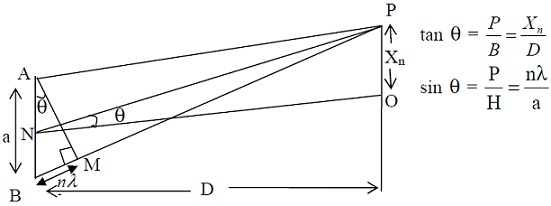In this section you will see that the thickness Y between two adjacent bright or dark fringes and a is the distance between the slits A and B. D is the distance of the slit from the screen and the wavelength of the monochromatic light is λ.

In Figure given above P is the position of nth bright fringe, then path difference at that point P is provided by

BP - AP = BM = nλ.

Consider a Δ NPO, then

tan Θ = Xn/D...........................................Eq.4

Similarly in Δ AMB,

sin Θ = nλ/a...........................................Eq.5

In practice, D is large and Xn is very small, therefore Θ is small,

Hence tan Θ ≈ sin Θ

Therefore Xn/D = nλ/a

Position of the nth bright fringe from origin O is provided by the relation

Or Xn = nλD/a................Eq.6

The distance of the next bright fringe from O is given by Xn + 1

Xn + 1 = (n+1)λD/a................Eq.7

[i.e. replaced n with n+1]

Hence the spacing Y between the nth and (n+1)th fringes can be determined by subtracting Eq.6 from Eq.7

Y = Xn+1 - Xn

Therefore on substituting values

Y = (n+1)λD/a - nλD/a

Therefore the expression for fringe width is

Y = λD/a ................Eq.8

Thus it can be found from Eq.8) that fringe width differs directly proportional to D and l and inversely proportional to distance between slits a. Therefore using expression in Eq. 8, one can estimate wavelength of light easily.

Tutorsglobe: A way to secure high grade in your curriculum (Online Tutoring)

Expand your confidence, grow study skills and improve your grades.

Since 2009, Tutorsglobe has proactively helped millions of students to get better grades in school, college or university and score well in competitive tests with live, one-on-one online tutoring.

Using an advanced developed tutoring system providing little or no wait time, the students are connected on-demand with a tutor at www.tutorsglobe.com. Students work one-on-one, in real-time with a tutor, communicating and studying using a virtual whiteboard technology.  Scientific and mathematical notation, symbols, geometric figures, graphing and freehand drawing can be rendered quickly and easily in the advanced whiteboard.

Free to know our price and packages for online physics tutoring. Chat with us or submit request at info@tutorsglobe.com